GRE Subject Test: Chemistry : Other Statistics

Example Questions

Example Question #272 : Gre Subject Test: Chemistry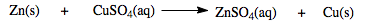Determine the percentage yield if the expected yield of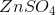in the above reaction is 40.911 grams but the actual yield is 35.322 grams.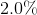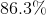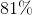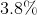Explanation:

In chemistry, percentage yield is a term used to quantify the efficiency of a chemical reaction. In order to calculate this quantity, the value for the actual yield and theoretical yield is needed. The actual yield is the amount of product obtained during a chemical reaction. The theoretical yield is the maximum amount of product possible to be obtain by a chemical reaction which is based on the amount of reactants used. Below is the formula needed to calculate the percentage yield.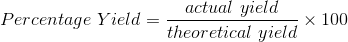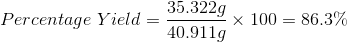Example Question #12 : Analyzing Data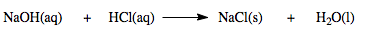0.0031 moles of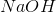was reacted with 0.0071 moles of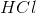giving an actual yield of 0.1733 grams of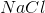. What is the percent yield of this reaction?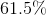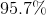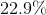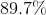Explanation:is the limiting reactant because based on the equation,andreact in a 1:1 mole ratio. However, there is 0.0040 moles more ofthanpresent. Therefore the amount ofproduced is limited by the amount ofpresent in the reaction. Based on the equation, for every 1 mole ofreacted, 1 mole ofwas produced: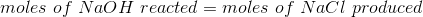Therefore,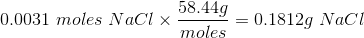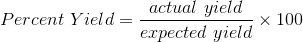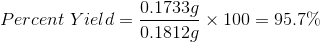Example Question #1 : Other Statistics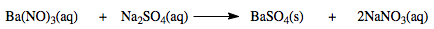Determine the percentage yield if the expected yield of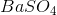in the above reaction is 1.2124 grams but the actual yield is 0.9749 grams.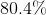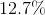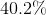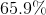Explanation:

In chemistry, percentage yield is a term used to quantify the efficiency of a chemical reaction. In order to calculate this quantity, the value for the actual yield and theoretical yield is needed. The actual yield is the amount of product obtained during a chemical reaction. The theoretical yield is the maximum amount of product possible to be obtain by a chemical reaction which is based on the amount of reactants used. Below is the formula needed to calculate the percentage yield.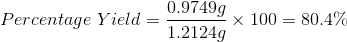All GRE Subject Test: Chemistry Resources